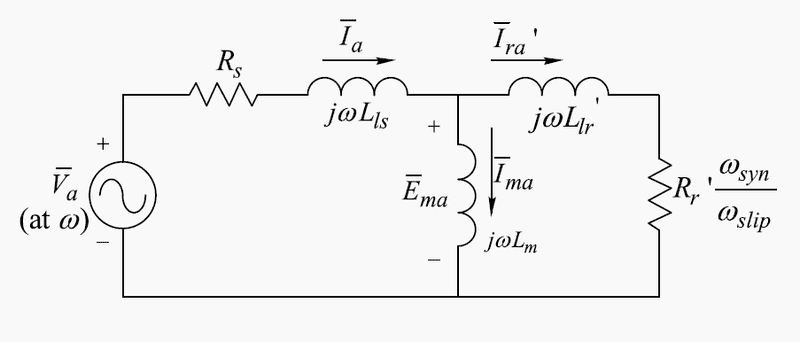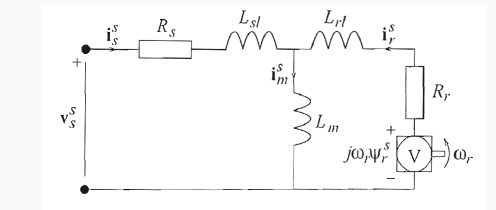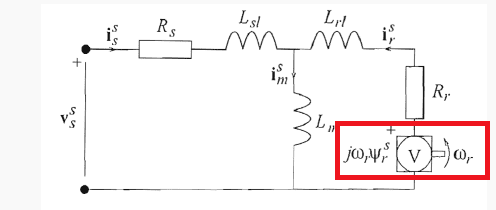# Equivalent circuit of an Induction Motor

Hi,
I have attached two circuits of an induction motor, one for steady state(1st circuit) and a dynamic model(2nd circuit).

My question is: Why don't we include the induced emf in the steady state circuit? Looking at the dynamic circuit we include it, but not in the steady state circuit. Is the induced emf somehow included in the following term $R_{r}^{'} \cdot \frac{\omega_{syn}}{\omega_{slip}}$ or is this somehow equal to $R_{r} + j \omega_{r} \Psi_{r}^{s}$ in steady state?

Please note that the superscripts $'$ and $^s$ means refereed to stator side.

If any additional information is required please let me know, and I will supply it as soon as possible.

EDIT1:
One more question came to mind, in this term: $j \omega_{r} \Psi_{r}^{s}$ why do we use $\omega_{rotor}$ instead of $\omega_{slip}$?
Since the induced emf and flux have the following relation $\varepsilon = -\frac{d \lambda}{dt}$ To my understanding I believe we should use the $\omega_{slip}$, because that is the relative motion of the rotor seen from the stator which magnetic flux $\Phi$ is rotating at frequency $\omega_{syn}$.Dynamic Circuit:Best Regards

Last edited:

The stator currents are regular a.c. currents produced by supplying Vst voltage in stator circuit. This current will produce an “in time variable” magnetic field and then an EMF opposing the supplying voltage.

The total variable- static- a.c. magnetic fields produced in each of three phases’ windings behaves as a rotated pole wheel. This common three_ phase rotating field will induce emf in the rotor. The rotor current frequency is direct proportionally with the slip frot=fstator*s.

The rotor resistance- never-the-less depends on rotor current frequency as skin effect and thermal effect ,heated by rotor current-could be considered constant. The rotor leakage field reactance depends directly on rotor frequency : Xrots=2*pi()*frot*Lroto=2*pi*f*s*Lroto Lroto=Lrot at s=1 [at start].[It is also an approximation since L depends also on s- for squirrel cage rotor mainly.]
In order to simplify the motor diagram, in the rotor circuit we may multiply the rotor resistance and rotor reactance by rotor winding to stator winding factors k^2 where k= kw2/kw1*n2/n1 and to divide the rotor resistance by s and so you can consider the rotor as stator extension and EMFrot/k =EMFst and V'r=Vr/k we get the current I'rot=Irot*k.
If we should neglect Rfe and Xm- as easier may consider at a transformer where the no-load current is about 2-3% of the rated but here the no-load current could be 20-30% -we could state Vst=Ist*[(Rs+R'r/s)+j*(Xs+X'r)].

#### Attachments

I do not think I understand it fully yet.

Looking at my image below, what does the element inside the red box represent?
My thought is that it represent the the induced emf ($\varepsilon=-\frac{d \lambda}{dt}$) in the rotor due to relative motion between the rotor squirrel cage and the rotating magnetic field set up by the stator, am I right?

If I am right, then why is the term $j\omega_{r}\Psi_{r}^{s}$ not included in the steady state equivalent circuit of the induction machine?Sorry for poor explanation, my background is not electrical, but I am currently learning it.

Best Regards

The dynamic circuit it is not actually a circuit but a symbolic "like" circuit.

The dynamic diagram is referring to space vector [all three phases in matrix form] and connected with Laplace transform [“p” or ” s” noted]. It facilitates to find the Laplace transform for a circuit where instead of derivate it will be p and instead of integral 1/p.

In order to find the actual values you have to integrate the result according to Laplace transform rules.

If you'll write the voltage drops on stator circuit and rotor circuit you’ll get:

Vs s = Rs Is s + p λs s stator circuit

0 = Rr*Ir s + (p - jωo) λr s rotor circuit where:

λs s = Ls Is s + Lm Ir s and λr s = Lm Is s + Lr Ir s

Since Laplace transform is an integral you have to consider the limits and the lower limit is rotor velocity [wo=wr].So jωo λr s= jωrΨs
See:
http://mathworld.wolfram.com/LaplaceTransform.html
http://www.drivetechinc.com/articles/IM98VC1.pdf
http://lipo.ece.wisc.edu/1994pubs/94-14T.pdf

•LagCompensator